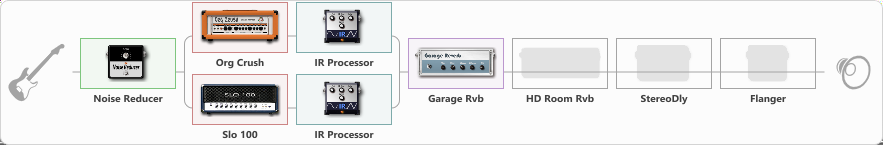# DISTROyer v.0667

Discussion in 'ToneLib-GFX presets' started by adamz667, Nov 6, 2022.

1. DISTROyer v.0667

Preset name: DISTroer V0.9

Hi gain preset for metal enthusiasts

Effects chain:Effect: "Noise Reducer" (Dynamics / Filter), active - "yes"
{
"Sens" = 50
"Mode" = Hard
}

Effect: "Splitter" (Dynamics / Filter)
{
"A-Bypass" = Off
"A-Pan" = -26
"A-Level" = 50
"B-Bypass" = Off
"B-Pan" = 24
"B-Level" = 75
"Width" = 0

'A' branch:
{

Effect: "Org Crush" (Amp simulators), active - "yes"
{
"Gain" = 75
"Bass" = 69
"Middle" = 50
"Treble" = 100
"Presence" = 85
"Master" = 61
"Level (dB)" = 0
}

Effect: "IR Processor" (Cabinets), active - "yes"
{
"IR" = Simpulse_ONE_BOTH
"Low Cut (Hz)" = 16
"Hi Cut (kHz)" = 20.0
"Mix" = 100
"Level (dB)" = 4
}
}
'B' branch:
{

Effect: "Slo 100" (Amp simulators), active - "yes"
{
"Gain" = 75
"Bass" = 64
"Middle" = 41
"Treble" = 74
"Presence" = 74
"Master" = 62
"Level (dB)" = 0
}

Effect: "IR Processor" (Cabinets), active - "yes"
{
"IR" = Pantera - VDOP - IR_dc
"Low Cut (Hz)" = 15
"Hi Cut (kHz)" = 20.0
"Mix" = 100
"Level (dB)" = 0
}
}
}

Effect: "Garage Rvb" (Reverberation), active - "yes"
{
"Time" = 3.0
"PreDelay" = 0
"LoDamp" = 5
"HiDamp" = 8
"Mix" = 9
}

Effect: "HD Room Rvb" (Reverberation), active - "no"
{
"Time" = 7.7
"PreLPF" = 100
"PreDelay" = 71
"HiDamp" = 55
"LoGain" = -3.5
"Mix" = 41
}

Effect: "StereoDly" (Delay), active - "no"
{
"Time" = 436
"Feedback" = 44
"Tone" = 76
"Sens" = 0
"Mix" = 68
}

Effect: "Flanger" (Modulation / Sfx), active - "no"
{
"Speed" = 1.6
"Depth" = 71
"Reso" = 35
"Center" = 7.7
"Offset" = 0.0
"Mix" = 75
"Reset" = On
}

Note: You will need to download and install the ToneLib-GFX software to use the preset.

#### Attached Files:

• ###### DISTROyer_v.tlgfx
File size:
24.7 KB
Views:
2,790
PAR3VAL likes this.